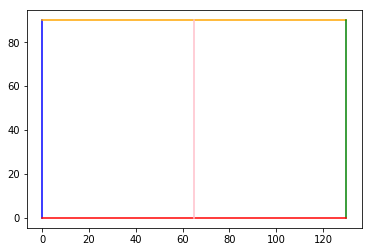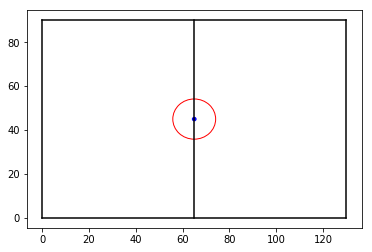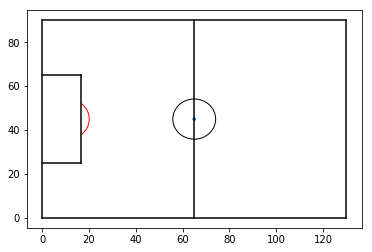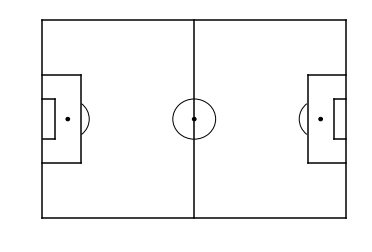# Drawing a Pitchmap – Adding Lines & Circles in Matplotlib

There are lots of reasons why we might want to draw a line or circle on our charts. We could look to add an average line, highlight a key data point or even draw a picture. This article will show how to add lines, circles and arcs with the example of a football pitch map that could then be used to show heatmaps, passes or anything else that happens during a match.

This example works with FIFA’s offical pitch sizes, but you might want to change them according to your data/sport/needs. Let’s import matplotlib as normal, in addition to its Arc functionality.

In :
```import matplotlib.pyplot as plt
from matplotlib.patches import Arc
```

### Drawing Lines

It is easiest for us to start with our lines around the outside of the pitch. Once we create our plot with the first two lines of our code, drawing a line is pretty easy with ‘.plot’. You have probably already seen ‘.plot’ used to display scatter points, but to draw a line, we just need to provide two lists as arguments and matplotlib will do the thinking for us:

• List one: starting and ending X locations
• List two: starting and ending Y locations

Take a look at the code and plot below to understand our outlines. Use the colour guides to see how they are plotted with start and end point lists.

In :
```fig=plt.figure()

plt.plot([0,0],[0,90], color="blue")
plt.plot([0,130],[90,90], color="orange")
plt.plot([130,130],[90,0], color="green")
plt.plot([130,0],[0,0], color="red")
plt.plot([65,65],[0,90], color="pink")

plt.show()
```Great job! Matplotlib makes drawing lines very easy, it just takes some clear thinking with start and end locations to get them plotted.

### Drawing Circles

Next up, we’re going to draw some circles on the pitch. Primarily, we need a centre circle, but we also need markers for the centre and penalty spots.

Adding circles is slightly different to lines. Firstly, we need to assign our circles to a variable. We use ‘.circle’ to do this, passing it two essential arguments:

• X/Y coordinates of the middle of the circle

For our circles, we’ll also assign colour and fill, but these are optional.

With these circles assigned then use ‘.patch’ to draw the circle to our plot.

Take a look at our code below:

In :
```#Create figure
fig=plt.figure()

#Pitch Outline & Centre Line
plt.plot([0,0],[0,90], color="black")
plt.plot([0,130],[90,90], color="black")
plt.plot([130,130],[90,0], color="black")
plt.plot([130,0],[0,0], color="black")
plt.plot([65,65],[0,90], color="black")

#Assign circles to variables - do not fill the centre circle!
centreCircle = plt.Circle((65,45),9.15,color="red",fill=False)
centreSpot = plt.Circle((65,45),0.8,color="blue")

#Draw the circles to our plot

plt.show()
```### Drawing Arcs

Now that you can create circles, arcs will be just as easy – we’ll need them for the lines outside the penalty area. While they take a few more arguments, they follow the same pattern as before. Let’s go through the arguments:

• X/Y coordinates of the centrepoint of the arc, assuming the arc was a complete shape.
• Width – we must pass width and height as the arc might not be a circle, it might instead be from an oval shape
• Height – as above
• Angle – degree rotation of the shape (anti-clockwise)
• Theta1 – start location of the arc, in degrees
• Theta2 – end location of the arc, in degrees

That’s a few more arguments than for the circle and lines, but don’t let that make you think that this is too much more complicated. Our code will look like this for one arc:

leftArc = Arc((11,45),height=18.3,width=18.3,angle=0,theta1=310,theta2=50)

All that we need to do after this is draw the arc to our plot, just like with the circles:

You can see this in action below:

In :
```#Demo Arcs

#Create figure
fig=plt.figure()

#Pitch Outline & Centre Line
plt.plot([0,0],[0,90], color="black")
plt.plot([0,130],[90,90], color="black")
plt.plot([130,130],[90,0], color="black")
plt.plot([130,0],[0,0], color="black")
plt.plot([65,65],[0,90], color="black")

#Left Penalty Area
plt.plot([16.5,16.5],[65,25],color="black")
plt.plot([0,16.5],[65,65],color="black")
plt.plot([16.5,0],[25,25],color="black")

#Centre Circle/Spot
centreCircle = plt.Circle((65,45),9.15,fill=False)
centreSpot = plt.Circle((65,45),0.8)

#Create Arc and add it to our plot
leftArc = Arc((11,45),height=18.3,width=18.3,angle=0,theta1=310,theta2=50,color="red")

plt.show()
```### Bringing everything together

The code below applies the above lines, cricles and arcs to a function for quick and easy use. The only new line removes our axes:

plt.axis(‘off’)

Take a look through our function belong and follow what we are doing. Feel free to take this and use it as the base for your own plots!

In :
```def createPitch():

#Create figure
fig=plt.figure()

#Pitch Outline & Centre Line
plt.plot([0,0],[0,90], color="black")
plt.plot([0,130],[90,90], color="black")
plt.plot([130,130],[90,0], color="black")
plt.plot([130,0],[0,0], color="black")
plt.plot([65,65],[0,90], color="black")

#Left Penalty Area
plt.plot([16.5,16.5],[65,25],color="black")
plt.plot([0,16.5],[65,65],color="black")
plt.plot([16.5,0],[25,25],color="black")

#Right Penalty Area
plt.plot([130,113.5],[65,65],color="black")
plt.plot([113.5,113.5],[65,25],color="black")
plt.plot([113.5,130],[25,25],color="black")

#Left 6-yard Box
plt.plot([0,5.5],[54,54],color="black")
plt.plot([5.5,5.5],[54,36],color="black")
plt.plot([5.5,0.5],[36,36],color="black")

#Right 6-yard Box
plt.plot([130,124.5],[54,54],color="black")
plt.plot([124.5,124.5],[54,36],color="black")
plt.plot([124.5,130],[36,36],color="black")

#Prepare Circles
centreCircle = plt.Circle((65,45),9.15,color="black",fill=False)
centreSpot = plt.Circle((65,45),0.8,color="black")
leftPenSpot = plt.Circle((11,45),0.8,color="black")
rightPenSpot = plt.Circle((119,45),0.8,color="black")

#Draw Circles

#Prepare Arcs
leftArc = Arc((11,45),height=18.3,width=18.3,angle=0,theta1=310,theta2=50,color="black")
rightArc = Arc((119,45),height=18.3,width=18.3,angle=0,theta1=130,theta2=230,color="black")

#Draw Arcs

#Tidy Axes
plt.axis('off')

#Display Pitch
plt.show()

createPitch()
```### Summary

In our article, we’ve seen how to draw lines, arcs and circles in Matplotlib. You’ll find this useful when trying to add the finishing touches with annotations to any plot. These tools are equally important when drawing a map on which we will plot our data – like our pitchmap example here.

Take a look at our other visualisation articles here and be sure to get in touch with us on Twitter!